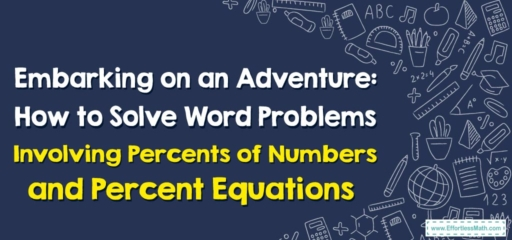# Embarking on an Adventure: How to Solve Word Problems Involving Percents of Numbers and Percent Equations

Today, we're going to venture into the realm of percent word problems. Just like the heroes in our favorite stories, we too shall encounter challenges – but fear not! With courage, determination, and a good understanding of percentages, we will surely succeed!## 1. Setting the Scene: What are Percents of Numbers and Percent Equations?

To prepare for our journey, we must first understand the tools at our disposal:

• Percents of Numbers: This is simply a part of the whole. If you have $$100$$$$\%$$ of something, you have the whole thing. If you have $$50$$$$\%$$, you have half. You get the idea!
• Percent Equations: These equations involve finding a certain percentage of a number, or determining what percent one number is of another.

## 2. The Quest: Solving Word Problems Involving Percents of Numbers and Percent Equations

Ready your mathematical swords and shields! It’s time to tackle our word problems.

### A Storyteller’s Guide: Solving Word Problems Involving Percents of Numbers and Percent Equations

#### Step 1: Understand the Problem

Just like deciphering a map, first, we must understand our problem. What are we trying to find? What information is given?

#### Step 2: Write the Equation

Now, we write an equation to represent our problem. If you’re finding a percent of a number, the equation might look like this: $$(\frac{percent}{100}) \times whole \ number= part$$.

#### Step 3: Solve the Equation

With our equation set, it’s time to solve it! Do the math and find your answer.

Once we’ve solved the problem, let’s check our answer. Does it make sense in the context of the problem?

Let’s say a magical shop is offering a $$30$$$$\%$$ discount on a wand that costs $$100$$. How much is the discount?

1. Understand the Problem: We’re trying to find out how much we’ll save with the discount.
2. Write the Equation: $$(\frac{30}{100}) \times 100 =\ discount$$
3. Solve the Equation: Our math gives us. $$30\times1=30$$
4. Check Your Answer: A $$30$$ discount on a $$100$$ wand? That makes sense!

Congratulations, brave adventurers! You’ve successfully navigated the world of percent word problems. But don’t rest yet! There are many more mathematical lands to explore and challenges to conquer. Onwards to the next adventure!

### What people say about "Embarking on an Adventure: How to Solve Word Problems Involving Percents of Numbers and Percent Equations - Effortless Math: We Help Students Learn to LOVE Mathematics"?

No one replied yet.

X
30% OFF

Limited time only!

Save Over 30%

SAVE $5 It was$16.99 now it is \$11.99